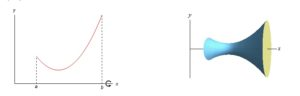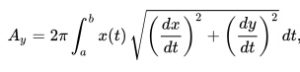# Surface of Revolution

A surface of revolution is the surface that you get when you rotate a two dimensional curve around a specific axis.

The image below shows a function f(x) over a closed interval [a, b], and the surface of revolution you get when you rotate it around the x axis:• If your line isn’t parallel to the axis it is rotated around, the surface of revolution will be a cone, or a part of a cone.
• If your curve is a straight line, parallel to the axis you are rotating around, your surface of revolution will be a cylinder.
• The surface of revolution of a line perpendicular to the axis will just be a circle.

## Finding the Area of a Surface of Revolution.

You can use calculus to find the area of a surface of revolution. Suppose the curve is described by two parametric functions x(t) and y (t); you want to find the surface that results when the segment of that curve ranging from x = a to x = b is rotated around the y axis. Then, so long as x(t) is not negative on the interval, the area of the surface you generate will be:This general formula can be specialized to situations you are likely to meet in math problem sets or in engineering applications. For example, if you have a curve represented by y = f(x), a ≤ x ≤ b, and you want to revolve around the x axis, you can simplify your surface area integral as:If you’re revolving around the y axis, on the interval a ≤ y ≤ b, the integral becomes:## Surface vs. Object of Revolution

In calculus 1, you used two methods to find volumes for objects of revolution:

1. Disc method: is a way to find the volume of solids of revolution.
2. Washer Method: a modification of the disc method to allow for objects with holes.Discs (left) and washers (right) are used to calculate volumes. The axis of rotation is the orange dashed line.

But what we’re interested in here isn’t the volume, but rather the surface. For example, instead of the volume of a whole orange, now we’re interested in the skin.

CITE THIS AS:
Stephanie Glen. "Surface of Revolution" From StatisticsHowTo.com: Elementary Statistics for the rest of us! https://www.statisticshowto.com/surface-of-revolution/